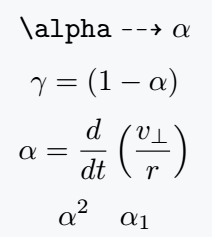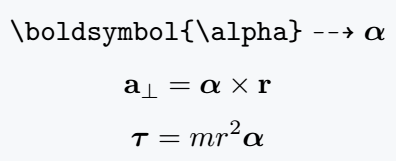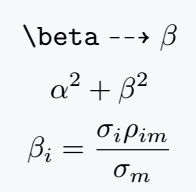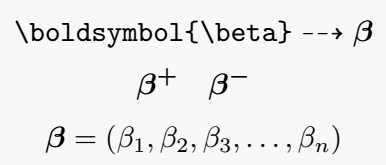# How to denote Alpha(α) and Beta(β) symbol in LaTeX?

The first two letters of the Greek alphabet are alpha and beta. In mathematics, these two symbols are used as variables.

In this tutorial, we will discuss how to write these two symbols in LaTeX.

## Alpha symbol in LaTeX

You do not need to use any package to print the alpha symbol in a LaTeX document.

Using \alpha, the default command of LaTeX, the symbol will be printed in the document, and it is used in LaTeX math mode.

So, you must place the command in single dollar $ or . Symbol Alpha Type of symbol Greek Letter Package No Argument No Latex command \alpha Example \alpha → α \documentclass{article} \usepackage{amssymb} \begin{document} $\verb|\alpha|\dashrightarrow \alpha$ $\gamma=(1-\alpha)$ $\alpha ={\frac{d}{dt}}\left({\frac{v_{\perp }}{r}}\right)$ $\alpha^2 \quad \alpha_1$ \end{document} Output :Also, to get the bold output of the alpha symbol, you need to use a package called amsmath. This package provides a command which is \boldsymbol{arg}. If you use the \alpha command as an argument for this command like \boldsymbol{\alpha}, the symbol will be printed in bold font in the document. \documentclass{article} \usepackage{amssymb} \usepackage{amsmath} \begin{document} $\verb|\boldsymbol{\alpha}|\dashrightarrow \boldsymbol{\alpha}$ $\mathbf{a}_{\perp}=\boldsymbol{\alpha}\times \mathbf{r}$ $\boldsymbol{\tau}=mr^{2}\boldsymbol{\alpha}$ \end{document} Output :## Beta symbol in LaTeX You do not need a package to write the beta symbol in Latex. Latex has a default command which is \beta, with this command you can print the symbol in a latex document. And it is also used in latex math mode, so you must place the command in single dollar $ or .

Symbol Beta
Type of symbol Greek Letter
Package No
Argument No
Latex command \beta
Example \beta → β
\documentclass{article}
\usepackage{amssymb}
\begin{document}
$\verb|\beta|\dashrightarrow \beta$
$\alpha^2 + \beta^2$
$\beta_i =\frac{\sigma_i \rho_{im}}{\sigma_m}$
\end{document}

Output :To get the bold output of the beta symbol, you need to use a package called amsmath. This package provides a command which is \boldsymbol{arg}.

If you use the \beta command as an argument for this command like \boldsymbol{\beta}, the beta symbol will be printed in bold font in the document.

\documentclass{article}
\usepackage{amssymb}
\usepackage{amsmath}
\begin{document}
$\verb|\boldsymbol{\beta}|\dashrightarrow \boldsymbol{\beta}$
$\boldsymbol{\beta^+} \quad \boldsymbol{\beta^-}$
$\boldsymbol{\beta}=(\beta_1,\beta_2,\beta_3,\dots,\beta_n)$
\end{document}

Output :#### Md Jidan Mondal

LaTeX expert with over 10 years of experience in document preparation and typesetting. Specializes in creating professional documents, reports, and presentations using LaTeX.